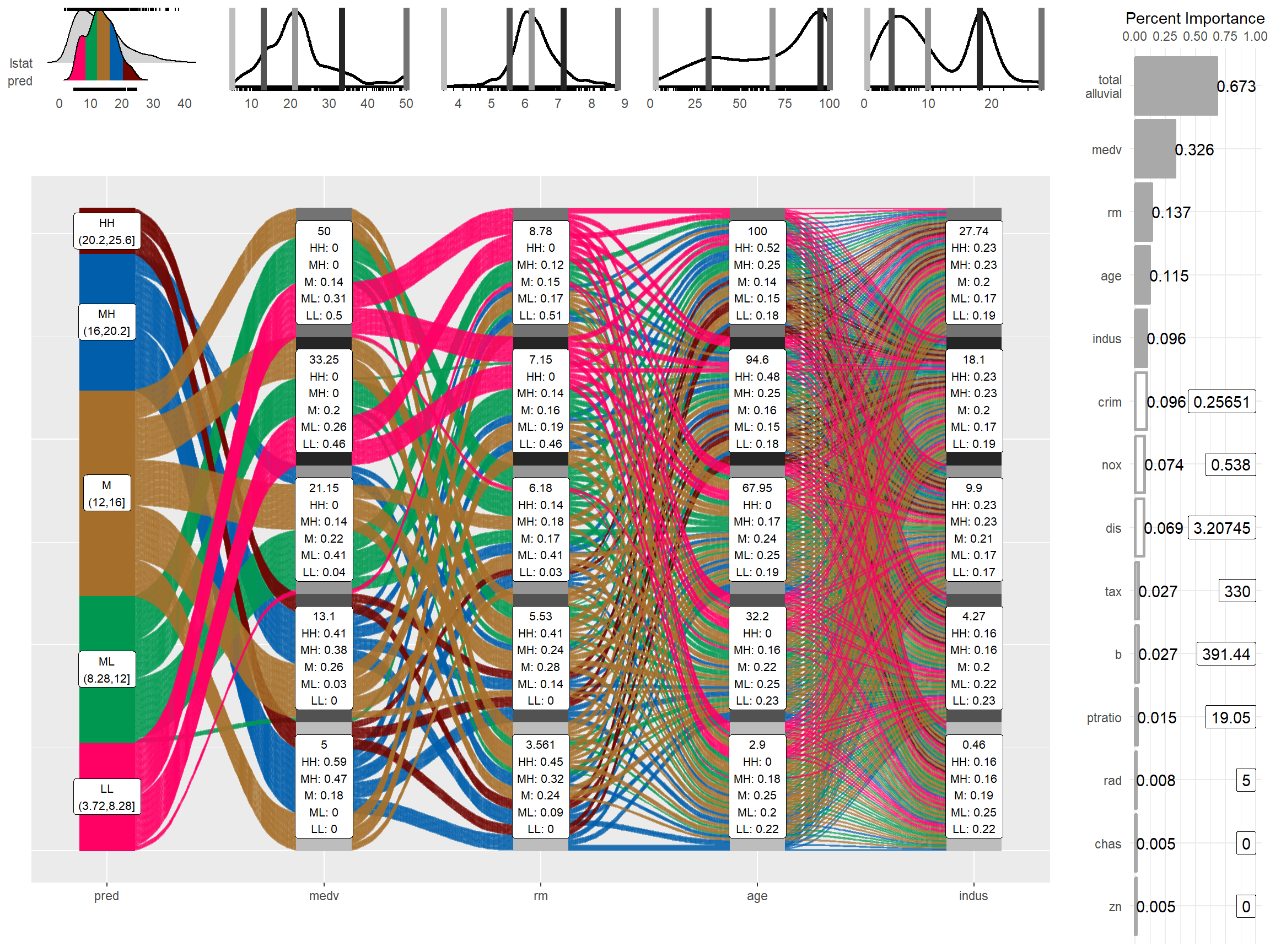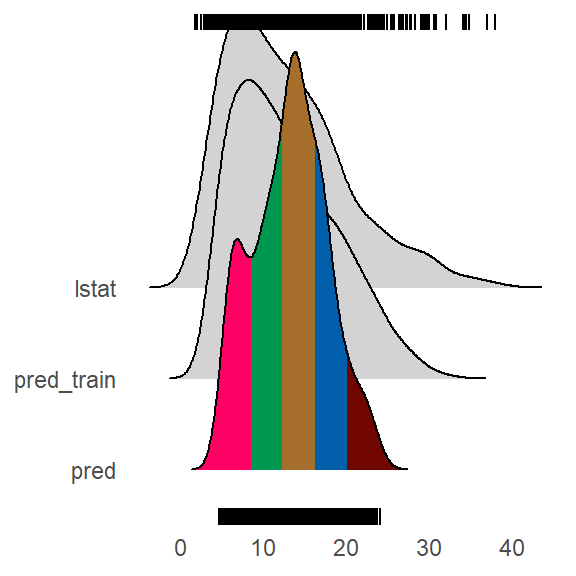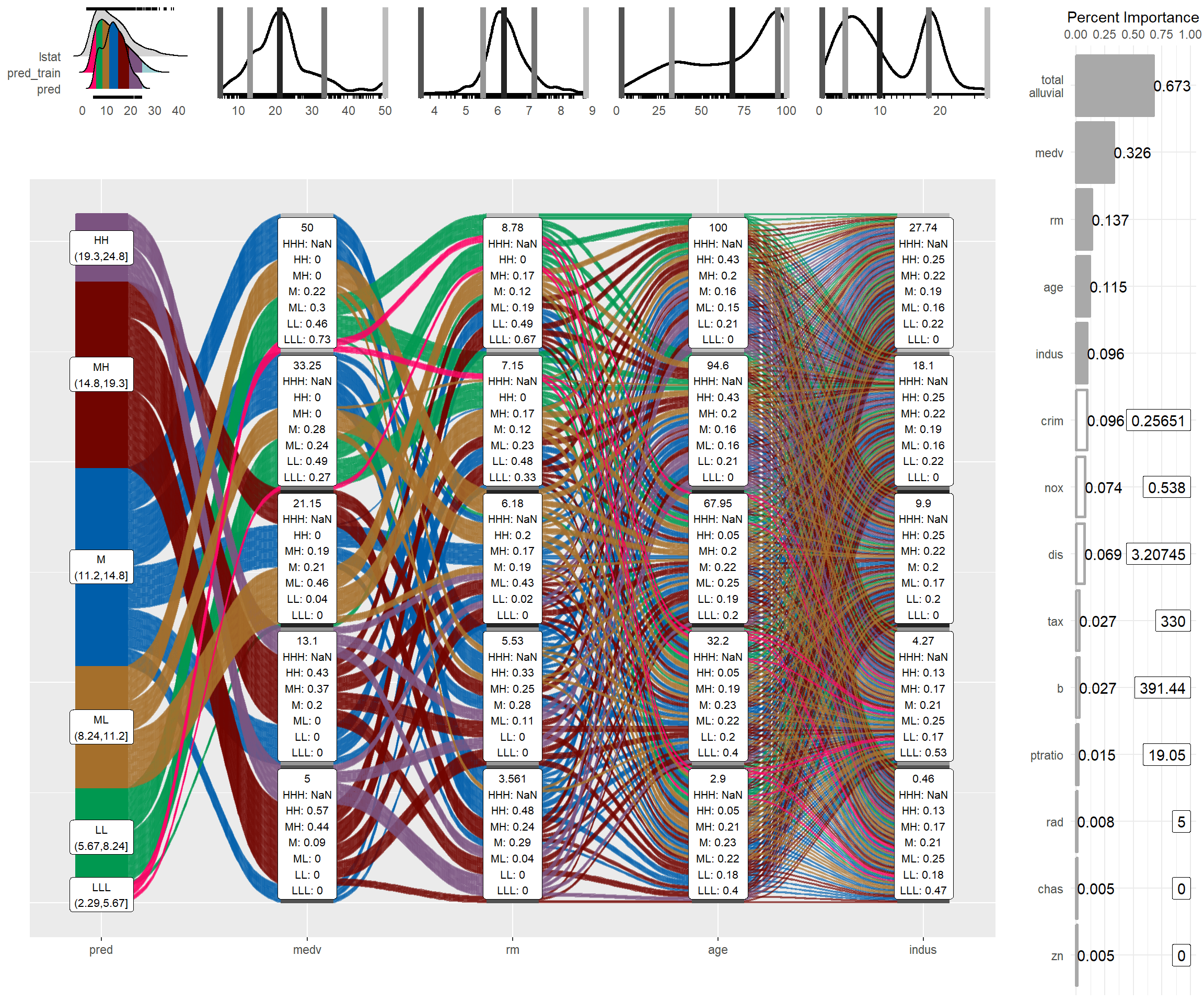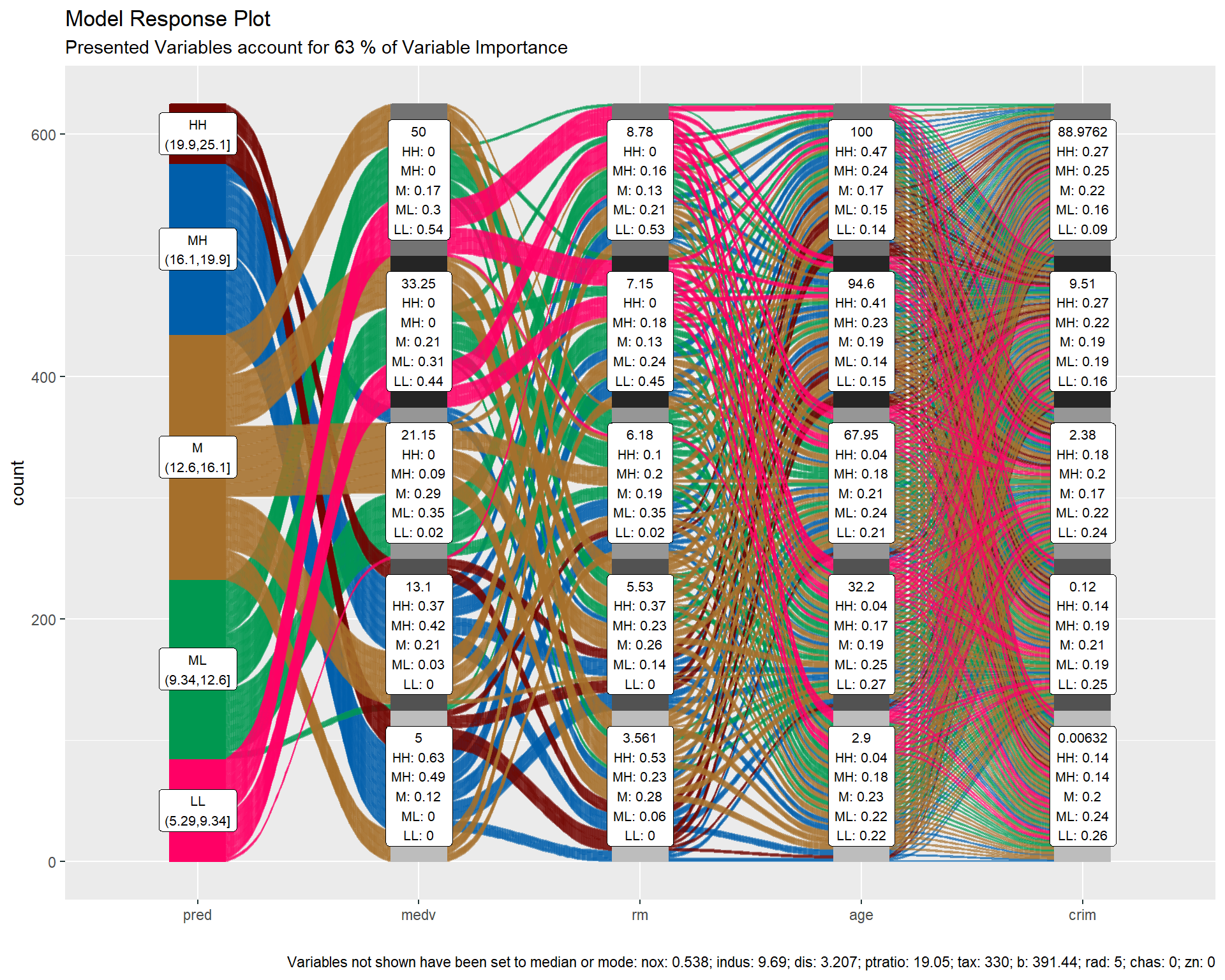Want to share your content on R-bloggers? click here if you have a blog, or here if you don't.

In this tutorial I want to show how you can use alluvial plots to visualise model response in up to 4 dimensions. `easyalluvial` generates artificial data space using fixed values for unplotted variables or uses the partial dependence plotting method. It is model agnostic but offers some convenient wrappers for `caret` models.

# Introduction

## Taking a peek

When building machine learning model we are usually faced with a trade-off between accurracy and interpretability. However even if we tend to lean towards accuracy and pick a modelling method that results in nearly uninterpretable models we can still make use of a bunch of model agnostic techniques that have been summarized in this excellent ebook Interpretable Machine Learning by Christoph Molnar.

Whithout getting to theoretical I personally always feel the urge to simply take a peek simulate some data and see how the model reacts a method described in Wickham H, Cook D, Hofmann H (2015) Visualizing statistical models: Removing the blindfold. Statistical Analysis and Data Mining 8(4) . In order to simulate data we can generate a a vector with a sequence of values over the entire range of a predictor variable of interest while setting all the others to their median or mode and use this artificial data space to obtain model predictions which we can plot against our variable of interest. An R package that will do this for you is (`plotmo`)[https://cran.r-project.org/web/packages/plotmo/index.html]. Instead of ranging over 1 predictor variable we can create a data grid using 2 predictor variables and plot the response as a third dimension. However this is as far as you can go in a conventional plots. Alluvial plots can line up much more than 3 dimensions on a plane next to each other only limited by the number of flows as it will get too cluttered when there are too many of them.

## Which variables to plot

When using conventional model response plotting beeing limited two 2 variables we can simply resolve this by generating many plots and look at them one by one. Alluvial plots require a bit more attention and cannot easily be screened and compared since visually there is so much going on. Therefore I do not recommend to brute force it by simply creating a lot of random combinations of predictor variables and multiple alluvial plots but instead to focus on those that have the highest calculated feature importance. Feature importance values are natively provided by most modelling packages. So the question is how many can we plot and it turns out 4 features will usually result in well interpretable plot.

## Generating the data space

```suppressPackageStartupMessages( require(tidyverse) )
suppressPackageStartupMessages( require(easyalluvial) )
suppressPackageStartupMessages( require(mlbench) )
suppressPackageStartupMessages( require(randomForest) )```

We start by creating a model

```data('BostonHousing')
df = as_tibble( BostonHousing )
m = randomForest( lstat ~ ., df )```

and looking at the importance features

```imp = m\$importance %>%
tidy_imp(df) #

knitr::kable(imp)```

vars imp
medv 8331.8325
rm 3554.7917
age 2697.7033
indus 2649.3554
crim 2306.9112
dis 1847.7221
nox 1547.4916
b 742.3811
tax 632.3429
ptratio 308.5192
zn 135.7454
chas 106.6607

When generating the data space we cannot screent and infinite amount of values per variable. We want to create all possible combinations between the values of the 4 variables we want to plot and an alluvial plot we cannot distinguish more than 1000 flows I recommend to go with 5 values which will result in 5 x 5 X 5 X 5 –> 625 combinations. That also leaves some wiggeling room if one of the top 4 variables is a factor with more than 5 levels. `get_data_space()` will split the range of a variable into 3 and picks the median of each split and add the variable minimum and the maximum to the set.

```dspace = get_data_space(df, imp
, degree = 4 # specifies the number of variables
, bins = 5 # the number of values per variable
)

medv rm age indus crim dis nox b tax ptratio rad zn chas
5 3.561 2.9 0.46 0.25651 3.20745 0.538 391.44 330 19.05 5 0 0
5 3.561 2.9 4.27 0.25651 3.20745 0.538 391.44 330 19.05 5 0 0
5 3.561 2.9 9.90 0.25651 3.20745 0.538 391.44 330 19.05 5 0 0
5 3.561 2.9 18.10 0.25651 3.20745 0.538 391.44 330 19.05 5 0 0
5 3.561 2.9 27.74 0.25651 3.20745 0.538 391.44 330 19.05 5 0 0
5 3.561 32.2 0.46 0.25651 3.20745 0.538 391.44 330 19.05 5 0 0
5 3.561 32.2 4.27 0.25651 3.20745 0.538 391.44 330 19.05 5 0 0
5 3.561 32.2 9.90 0.25651 3.20745 0.538 391.44 330 19.05 5 0 0
5 3.561 32.2 18.10 0.25651 3.20745 0.538 391.44 330 19.05 5 0 0
5 3.561 32.2 27.74 0.25651 3.20745 0.538 391.44 330 19.05 5 0 0

Total rows in dspace: 625

```dspace %>%
summarise_all( ~ length( unique(.) ) ) %>%
knitr::kable()```

medv rm age indus crim dis nox b tax ptratio rad zn chas
5 5 5 5 1 1 1 1 1 1 1 1 1

## Generating model response

`pred = predict(m, newdata = dspace)`

## Plotting

The predictions will be binned as well into 5 bins. Binning options can be passed as a list via the `params_bin_numeric_pred` parameter.

```p = alluvial_model_response(pred, dspace, imp
, degree = 4, bins = 5
, stratum_label_size = 2.8 )
p```We can seet the binned predictions and by which variable combination they have been created by tracing the coloured flows. The stratum labels of the prediction variables indicate the value of the variable and which fraction of the flows of that colour (prediction variable bin range) pass through that stratum.

## Marginal histograms

As well as for other `easyalluvial` plots we can add marginal histograms and as a bonus also the feature importance.

```p_grid = add_marginal_histograms(p, data_input = df
, plot = F # plot only after adding feature importance
, scale = 50 # to increase distance between ridge plots, Default: 400
) %>%
add_imp_plot( p = p, data_input = df)```We can see the original distribution of the variables and the lines indicate the position of the values as picked by `get_data_space()`. When comparing the distribution of the predictions against the original distribution of `lstat` we see that the range of the predictions in response to the artificial dataspace do not cover all of the range of `lstat`. Which most likely means that all possible combinations of the 4 plotted variables in combination with moderate values for all other predictors will not give any extreme values. But first we will need to check whether the model is capable of making predictions in the lower and upper ranges of `lsat`. We can use `plot_hist()` to only plot the distributions and add the prediction for the training data set using the `pred_train` parameter.

```pred_train = predict(m)

plot_hist('pred', p, df
, pred_train = pred_train # pred_train can also be passed to add_marginal_histograms()
, scale = 50)```We see that the training prediction also do not completely cover all of `lstat` range but more of it than the predictions from the aritficial data space.

If we wanted to emphasize this we can bin the data space predictions on the basis of the training predictions. In this case it makes sense to increase the number of bins for the predictions in order not to loose resolution on the plot.

```p = alluvial_model_response(pred, dspace, imp, degree = 4, bins = 7
, bin_labels = c("LLL","LL", "ML", "M", "MH", "HH", "HHH")
, pred_train = pred_train
, stratum_label_size = 2.8 )

plot_hist('pred', p, df, scale = 50)``````p_grid = add_marginal_histograms(p, data_input = df
, plot = F # plot only after adding feature importance
, scale = 50 # to increase distance between ridge plots, Default: 400
) %>%
add_imp_plot( p = p, data_input = df)```# What feature combinations are needed to obtain predictions in the lower and higher ranges of `lstat`?

We can add the training predictions to the training data and assign the variables not covered by the model response plot to bins. We can then create an alluvial plot over the entire training dataframe. Since we are only interested in those observations from the training data that cause predictions not covered by the model response plot we remove all other flows from the plot by setting their color to `NA`.

```breaks = c( min(pred_train) - 1,min(pred),max(pred),max(pred_train) + 1 )

df_inv = df %>%
select(-lstat) %>%
mutate( pred_train = pred_train
, pred_train = cut(pred_train, breaks) ) %>%
select( pred_train, one_of(imp\$vars) )

p = alluvial_wide(df_inv, bin_labels = 'min_max'
, stratum_label_size = 3
, col_vector_flow = c('blue', NA, 'orange')
, colorful_fill_variable_stratum = F)# `caret` wrapper

`caret` provides a uniformous interface for training and calling a lot of machine learning models as well as for calculating feature importance. `easyalluvial` provides a function that wraps the above described workflow into one single call. Note that feature importance values are slightly different. `randomForest` returns a combined importance for all levels of a factor variable while `caret` splits them up. `tidy_imp()` aggregates them and uses the maximum value of all values. This behaviour can be adjusted by passing another aggregating function to the `.f` parameter.

```train = caret::train( lstat ~ .
, df
, method = 'rf'
, trControl = caret::trainControl( method = 'none' )
, importance = TRUE )

alluvial_model_response_caret(train, degree = 4, bins = 5
, stratum_label_size = 2.8)```Model response alluvial plots can help us to get an immediate intuitive understanding how predictions of a certain range can be generated by the model. They can be understood by none-statistical stakeholders and invite the viewer to start exploring and question the decision making process of the model while also convey an appreciation for the model complexity as flows branch out to the variables of lower feature importance.

# Partial Dependence Plotting Method

1. construct the data space as described above, but instead of setting all none-plotted variables to median/mode we set them to the values found in the first row of the training data.

2. Repeat 1. for each row

3. Use all data spaces to get model predictions

4. Average model predictions

`get_pdp_predictions()` will do this for us, however we need to provide it with the prediction function appropriate for the type of the model. Usually those functions are not exported by the package but can be found using `:::`.

```pred = get_pdp_predictions(df, imp, m, degree = 4, bins = 5)

alluvial_model_response(pred, dspace, imp, degree = 4
, method = 'pdp' # changes title and caption
, stratum_label_size = 2.8 )```thanks to the uniformous modelling interface of `caret` `alluvial_model_response_caret()` can call `get_pdp_predictions()` directly.

```alluvial_model_response_caret(train, degree = 4, bins = 5
, method = 'pdp'
, stratum_label_size = 2.8 )```## A note on feature importance

Calculating feature importance in a dataset with strongly correlating variables will lead to inacurrate results. It usually means that two strongly correlating variables share the importance that would be accredited to them if only one of them was present in the data set. Further some feature importance calculation methods require the model to be trained with scaled, centered and potentially normalized (transformed to be more normally distributed) numerical variables in order to deliver meaningful results. We can use the `recipes` package to perform these tasks. Using the below described workflow we can create the artifical dataspace with untransformed numerical values which will give us more meaningful output.

```suppressPackageStartupMessages( require(recipes) )

# filter correlating variables recipe- ------------------------------
# caret tends to complain if recipe filters any variables, therefore
# we use two recipes

rec_filt_corrs = recipe(df, lstat ~ . ) %>%
step_corr( all_numeric()
, - all_outcomes()
, threshold = 0.7 ) %>%
prep()

df_filt_corrs = bake(rec_filt_corrs, df)

# transformation recipe --------------------------------------------
rec_trans = recipe( df_filt_corrs, lstat ~ .) %>%
step_center( all_numeric(), - all_outcomes() ) %>%
step_scale( all_numeric(), - all_outcomes() ) %>%
step_YeoJohnson( all_numeric(), - all_outcomes() ) %>%
prep()

# train and plot ---------------------------------------------------
set.seed(1)
train = caret::train( rec_trans
, df_filt_corrs
, method = 'earth'
, trControl = caret::trainControl( method = 'cv'
, search = 'random')
, tuneLength = 100)

pred_train = predict(train, df_filt_corrs)

p = alluvial_model_response_caret(train, degree = 4, bins = 5
, pred_train = pred_train
, stratum_label_size = 2.8 )

p_grid = add_marginal_histograms(p, df, plot = F
, pred_var = 'lstat'
, scale = 50) %>%## Limitations

• There is a loss of information when binning the numerical variables
• The combinations generated when making the grid might be outside the feature distribution space (generate combinations that are impossible)
• We only look at the combination of 4 features and disregard the others

To alleviate this you can reduce the complexity of the model by reducing features (take out correlating variables) or use additional model exploration methods such as classical PDPs, ALE plots, Shapely values, etc, …